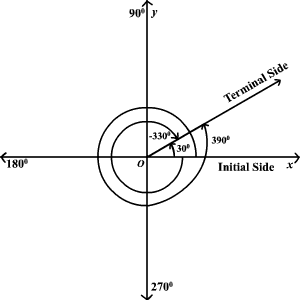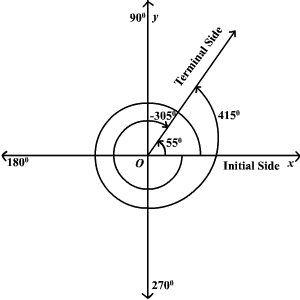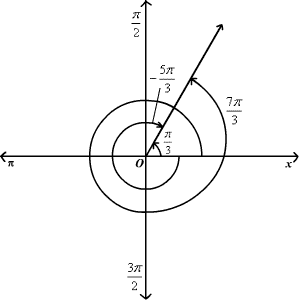# Coterminal Angles

Coterminal angles are angles in standard position (angles with the initial side on the positive $x$ -axis) that have a common terminal side. For example, and are all coterminal.To find a positive and a negative angle coterminal with a given angle, you can add and subtract if the angle is measured in degrees or  if the angle is measured in radians.

Example 1:

Find a positive and a negative angle coterminal with a angle.

angle and a angle are coterminal with a angle.Example 2:

Find a positive and a negative angle coterminal with a  angle.

angle and a  angle are coterminal with a angle.Find four coterminal angles of the following: Draw the coterminal angle with the original angle 210º -72º 510º Key questions to consider: Have you tried finding coterminal angles in both positive and negative directions? What is the same between the coterminal and original angles? What is different between the coterminal and original angles? How many times coterminal angles are possible? What applications can you use coterminal angles? For example, think of car tire rotating more than one time around.